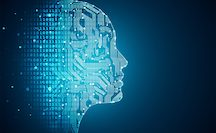# Pennsylvania State UniversityData Science for Researchers and ScholarsTexts and References

Primary (Required) Textbook

1. Shah, Chirag (2020). A Hands-On Introduction to Data Science, Cambdridge University Press
2. Skiena, S. (2017). Data Science Design Manual, Springer. Available for download by Penn State Students.

Recommended References

1. Daume, Hal (2017). A course in machine learning Freely available for download online.
2. Watt, J., Borhani, R., Katsagellos, A. (2020). Machine Learning Refined. Cambridge University Press. Available through Penn State Libraries here.

3. Deisenroth, M.P., Faisal, A., and Ong, C.S. (2018) Math for Machine Learning Cambridge University Press. Available through Penn State Libraries here.

4. Behrman, K. (2022). Foundational Python for Data Science.

5. Vanderplas, J. (2017). Python Data Science Handbook. O'Reilly. Freely available for online reading.

6. Chen, D. Y. (2018). Pandas for everyone. Pearson.

An annotated list of machine learning books

1. Abu-Mostafa, Y., Magdon-Ismail, M., and Lin, H-T. (2012). Learning from Data. AMLBook.com
A concise yet rigorous introduction to machine learning.

2. Baldi, P. and Brunak, S. (2002). Bioinformatics: A Machine Learning Approach. Cambridge, MA: MIT Press.
This book offers a good coverage of machine learning approaches - especially neural networks and hidden Markov models in bioinformatics.

3. Baldi, P., Frasconi, P., Smyth, P. (2003). Modeling the Internet and the Web - Probabilistic Methods and Algorithms. New York: Wiley.
A good introduction to machine learning approaches to text mining and related applications on the web.

4. Barber, D. (2012). Bayesian Reasoning and Machine Learning. Cambridge University Press.
An online text on machine learning, with emphasis on graphical models.

5. Bishop, C. M. Neural Networks for Pattern Recognition. New York: Oxford University Press (1995).
This book offers a good coverage of neural networks

6. Bowles, M. (2015). Machine Learning in Python: Essential Techniques for Predictive Analysis. Wiley.
A hands-on intro to some of the machine learning methods.

7. Chakrabarti, S. (2003). Mining the Web, Morgan Kaufmann.
Good coverage of machine learning applied to web mining.

8. Cohen, P.R. (1995) Empirical Methods in Artificial Intelligence. Cambridge, MA: MIT Press.
This is an excellent reference on experiment design, and hypothesis testing, and related topics that are essential for empirical machine learning research.

9. Courville, A., Goodfellow, I., and Bengio, Y. (2015). Deep Learning.
Online text on deep learning.

10. Cowell, R.G., Dawid, A.P., Lauritzen, S.L., and Spiegelhalter,D.J. (1999). Graphical Models and Expert Systems.Berlin: Springer.

This is a very good introduction to probabilistic graphical models.

11. Cristianini, N. and Shawe-Taylor, J. (2000). An Introduction to Support Vector Machines. London: Cambridge University Press.
This is an excellent introduction to kernel methods for pattern classification.

12. Devroye, Luc, Györfi, László, Lugosi, Gabor. (1996). A probabilistic theory of pattern recognition. Springer.
Excellent mathematically rigorous coverage of machine learning for pattern recognition.

13. Duda, R., Hart, P., and Stork, D. (2001). Pattern Classification. New York: Wiley.
A classic, albeit somewhat dated, text on statistical methods for pattern classification.

14. Hastie, T., Tibshirani, R., and Friedman, J. (2001). The elements of Statistical Learning - Data Mining, Inference, and Prediction. Berlin: Springer-Verlag.
This is an excellent text that explains some of the key ideas in machine learning from a statistical perspective.

15. Leskovec, U., Rajaraman, A., and Ullman, J. (2014). Mining Massive Data Sets
Online text focusing on mining large data sets

16. Kearns, M. and Vazirani, U. (1994). Computational Learning Theory. Cambridge, MA: MIT Press.
An excellent, albeit a bit dated, introduction to theoretical aspects of machine learning.

17. Koller, D. and Friedman, N. (2009). Probabilistic Graphical Models. MIT Press.

18. Mitchell, T. (1997). Machine Learning. New York: Mc Graw-Hill.
An excellent, albeit somewhat dated, introduction to Machine Learning.

19. Mohri, M., Rostamzadeh, A., and Talwalker, A. (2012). Foundations of Machine Learning MIT Press, 2012.
A rigorous introduction to machine leanring.

20. Murphy, K. (2012). Machine Learning: A probabilistic perspective. MIT Press.
An accessible survey of machine learning from a probabilistic perspective.

21. Natarjan, B. (1991). Machine Learning: A Theoretical Approach. Kluwer.
An excellent, albeit somewhat dated, text on theoretical aspects of machine learning.

22. Neapolitan, R. (2004). Learning Bayesian Networks. Prentice-Hall.

23. Rogers, S., and Girolami, M. (2016). First Course in Machine Learning. CRC Press.
A rigorous treatment of modern machine learning methods.

24. Russel, S. and Norvig, P. (2003). Artifiical Intelligence: A Modern Approach. 2nd Edition. New York: Prentice-Hall.
An excellent text on Artificial Intelligence, with several introductory chapters on Machine Learning.

25. Skolkopf, B. ad Smola, A. (2001). Learning with Kernels. MIT Press.
Excellent coverage of kernel methods in machine learning.

26. Tan, P-N., Steinbach, M., and Kumar, V. (2004). Introduction to Data Mining. New York: Addison-Vesley.
A good coverage of machine learning from a data mining perspective.

27. Theodoridis, S. (2015). Machine Learning. Springer.
A bayesian and optimization perspective on machine learning.

28. Vapnik, V. (1998). Statistical Learning Theory. Wiley.
An excellent coverage of structural risk minimization approach to machine learning.

29. Vidyasagar, M. (2002). A theory of learning and generalization, with applications to Neural Networks. Springer.
An excellent book covering the theoretical foundations of machine learning and generalization.

30. Watt, J., Borhani, R., Katsagellos, A. (2020). Machine Learning Refined. Cambridge University Press.
An excellent, mathematically rigorous, introduction to modern machine learning.

31. James, G., Witten, D., Hastie, T., and Tibshirani, R. (2014) An introduction to statistical learning: with application in R, Springer. Freely available for download online.